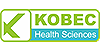ISSN 0886-3067Volume No 35 Issue No 1

Comparative Analysis Between Pre-test/Post-test Model and Post-test-only Model in Achieving the Learning Outcomes

Purpose: To compare the effectiveness of pre-test/post-test model with post-test-only model in achieving the learning outcomes of a lecture of 45 minutes. Study Design: Quasi experimental study. Study Place and Study Period: Rashid Latif Medical College, in 2018. Sample Collection: Non-probability convenience sampling. Material and Methods: 131 students of fourth year MBBS in a private medical college of Pakistan were selected for the study. Students of the same year and same college were included in the study. Two teaching models were compared on the same set of students at different time-periods. Pre-test followed by post-test model was compared with post-test-only model. In the pre-test/post-test design, the students were given a test before the lecture was delivered. The same test was given after a lecture of 45 minutes. The same group of students were taught another topic in another lecture of 45 minutes. There was no pre-test this time. 50 percent score was selected as the passing criteria. Results: There were 131 students, 66 males and 65 females (ratio of 1.01:1). In the pre-test/post-test model, 82% (n = 107) students passed the test while 5% (n = 6) failed. 14% (n = 18) students scored borderline marks. In the post-test-only model, 57% (n = 74) students passed the test while 6% (n = 8) failed. 37% (n = 49) students scored borderline marks. Conclusion: Pre-test/post-test model was significantly more effective in students in achieving the learning outcomes in a lecture as compared to post-test only model (p < 0.01). Key Words: Pre-test Post-test teaching model, Post-test-only design, Evaluation.